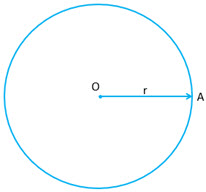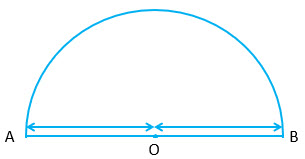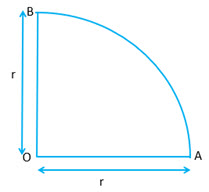a) If$r$$r$ is the radius of a circle then:$\displaystyle \text{ Perimeter } = 2 \pi r$$\displaystyle \text{ Perimeter } = 2 \pi r$$\displaystyle \text{Area } = \pi r^2 \text{ or Area } = \frac{1}{4} \pi d^2$$\displaystyle \text{Area } = \pi r^2 \text{ or Area } = \frac{1}{4} \pi d^2$$\displaystyle \text{Diameter } (d) = 2r$$\displaystyle \text{Diameter } (d) = 2r$b) If$r$$r$ is the radius of a circle then:$\displaystyle \text{Perimeter of a semi circle } = \pi r + 2r = (\pi + 2)r$$\displaystyle \text{Perimeter of a semi circle } = \pi r + 2r = (\pi + 2)r$$\displaystyle \text{Area of semi circle } = \frac{1}{2} \pi r^2$$\displaystyle \text{Area of semi circle } = \frac{1}{2} \pi r^2$c) If$r$$r$ is the radius of a circle then:$\displaystyle \text{Perimeter of the quadrant } = \frac{1}{4} \times 2 \pi r + 2 r = ( \frac{\pi}{2} + 2)r$$\displaystyle \text{Perimeter of the quadrant } = \frac{1}{4} \times 2 \pi r + 2 r = ( \frac{\pi}{2} + 2)r$$\displaystyle \text{Area of the quadrant } = \frac{1}{4} \pi r^2$$\displaystyle \text{Area of the quadrant } = \frac{1}{4} \pi r^2$d) If$R$$R$ and$r$$r$ are radii of two concentric circles, then$\displaystyle \text{ Area enclosed between the two circle } \\ \\ = \pi R^2 - \pi r^2 = \pi (R^2 - r^2) = \pi (R+r)(R-r)$$\displaystyle \text{ Area enclosed between the two circle } \\ \\ = \pi R^2 - \pi r^2 = \pi (R^2 - r^2) = \pi (R+r)(R-r)$e) If$\triangle ABC$ is an equilateral triangle of side$\displaystyle a \text{ and height } h \text{ , then } h = \frac{\sqrt{3}}{2} a$.

Also if$R$ and$r$ are the radii of the circumscribed and inscribed circles of$\displaystyle \triangle ABC$, then$\displaystyle R = \frac{2h}{3} \text{ and } r= \frac{h}{3}$$\displaystyle \text{Circumference of the circumcircle of } \triangle ABC = 2\pi R = 2 \pi \times \frac{2h}{3} + \frac{4}{3} \pi h$$\displaystyle \text{Area of the circumcircle } = \pi R^2 = \pi ( \frac{2h}{3} )^2 = \frac{4}{9} \pi h^2$$\displaystyle \text{Circumference of the inscribed circle } = 2 \pi r = \frac{2 \pi}{3} h$$\displaystyle \text{Area of the inscribed circle } = \pi r^2 = \frac{1}{9} \pi h^2$

f) Important notes:

• If two circles touch internally, then the distance between their centers is equal to the difference of their radii.
• If two circles touch externally. Then the distance between their centers is equal to the sum of their radii.
• The distance moved by a rotating wheel in one revolution is equal to the circumference of the wheel.
• The number of revolutions completed by a rotating wheel in one minute$\displaystyle = \frac{\text{Distance moved in one minute}}{\text{circumference}}$